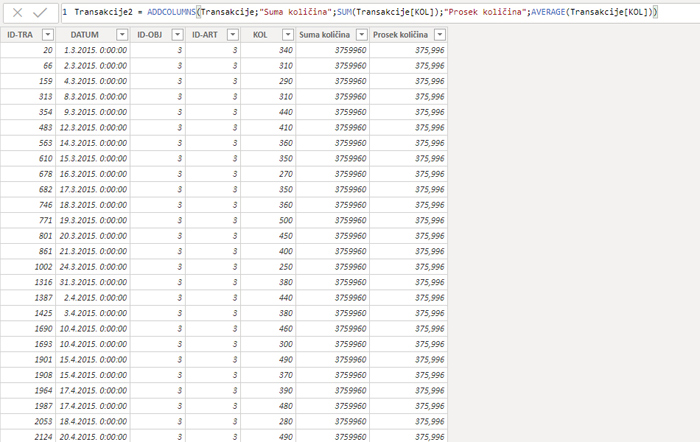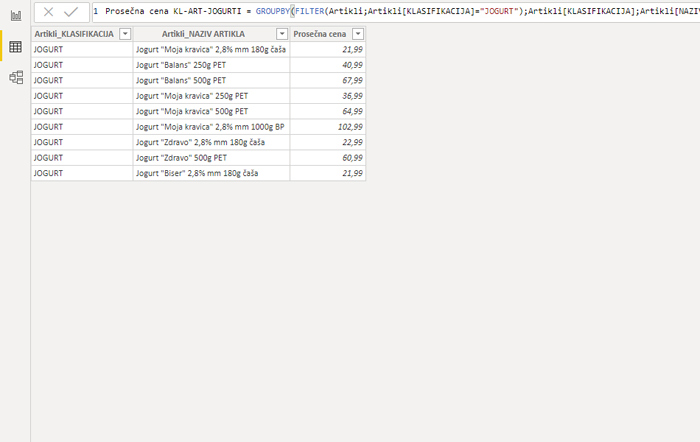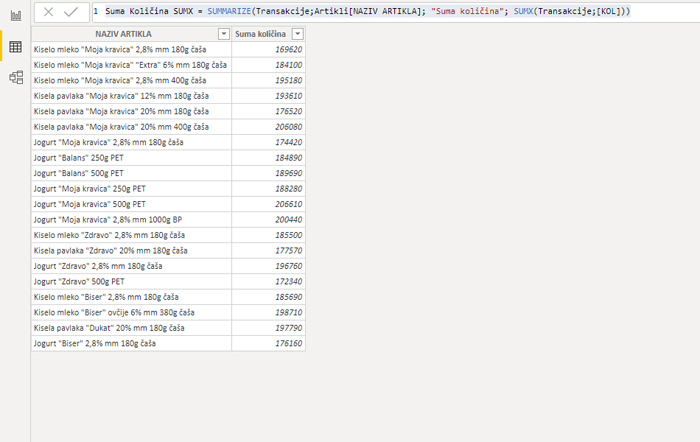# DAX table generating functions

DAX is a language that comes in several flavors. While most features are available at the same time for Excel, Power BI and SQL Server Analysis Services some of them are platform-specific. Because Power BI has the ability to generate tables and there are DAX functions that, as a result, return tables in this post we will address some of them that can only be only used in Power BI DAX statements, not in Excel.

We launched Power BI Desktop. After importing and linking several tables, we can create a new one by entering Data View, then selecting Modeling ribbon and New Table option. Then we should enter the name of the new table, followed by the expression on which it is created. Let’s see all of the table generating functions that exist and how we can use them!

ADDCOLUMS is a DAX function that adds columns to an existing table. The result is an existing table expanded for columns added. E.g. if we have a table Transakcije consisting of columns ID-TRA, DATUM, ID-OBJ, ID-ART and KOL, and we want to add the sum and average of all quantities sold we would write the expression:

“Prosek količina”;AVERAGE(Transakcije[KOL]))GROUPBY (<table>;[<groupBy_columnName1>];[<name>;<expression>]… )

GROUPBY is a DAX function that returns as a result a table that was created by selecting from one or more tables one or more columns by which to group, and then adding an expression that performs data aggregation. The name is required before the expression, which is actually a brief textual description of the column over which the aggregation was performed. We use some “X” function to calculate the expression, where the first parameter is the CURRENTGROUP() function, which indicates that the aggregation is performed over the data from the current group. E.g. to calculate the average price by classification and articles, the following expression should be written:

Prosečna cena KL-ART = GROUPBY(Artikli;Artikli[KLASIFIKACIJA];Artikli[NAZIV ARTIKLA];
“Prosečna cena”;AVERAGEX(CURRENTGROUP();Artikli[PC]))

How would we get a similar table in which grouping was done only for items where CLASSIFICATION = “JOGURT”? Well, instead of the first argument, which is a table name, you should specify a FILTER function that returns a filtered table for a given classification:

Prosečna cena KL-ART-JOGURTI = GROUPBY(FILTER(Artikli;Artikli[KLASIFIKACIJA]=”JOGURT”);Artikli[KLASIFIKACIJA];
Artikli[NAZIV ARTIKLA];”Prosečna cena”;AVERAGEX(CURRENTGROUP();Artikli[PC]))SUMMARIZE (<table>;<groupBy_columnName>[;<groupBy_columnName>]…[;<name>;<expression>]…)

SUMMARIZE is a DAX function that returns a table and consists of multiple columns by which groupings are made (they can be from different tables that we have previously linked in the Data Model), and displays one or more results that are obtained by calculating expressions. Similar to the GROUPBY function, the name that describes it must be specified before the expression. SUMMARIZE is a very liberal function because we can use different functions to compute statements. E.g. if we wanted to use the SUM function to calculate the sum of quantities by item names we would write a statement:

Suma Količina SUM = SUMMARIZE(Transakcije;Artikli[NAZIV ARTIKLA]; “Suma količina”; SUM(Transakcije[KOL]))

If we want, we can also use some “X” function, e.g. SUMX:

Suma Količina SUM = SUMMARIZE(Transakcije;Artikli[NAZIV ARTIKLA]; “Suma količina”; SUM(Transakcije[KOL]))

And we can also use everyone’s favorite CALCULATE function:

Suma Količina CALCULATE = SUMMARIZE(Transakcije;Artikli[NAZIV ARTIKLA];
“Suma količina”; CALCULATE(SUM(Transakcije[KOL])))

What would an old Serbian song say: “Here comes a joy to my heart”! Do you know what I mean?SUMMARIZECOLUMNS (<groupBy_columnName> [;< groupBy_columnName >]…;
[<filterTable>]…[; <name>;<expression>]…)

SUMMARIZECOLUMNS is a function that works similar to SUMMARIZE by first specifying a column, then a table, a name, and finally an expression. As with many previous cases, choosing which DAX function to use is all a matter of style. E.g. if we want to calculate the average price of yogurt, only for the value of the classification “JOGURT” we would write the statement:

Suma Količina SC = SUMMARIZECOLUMNS(Artikli[KLASIFIKACIJA];FILTER(Artikli;Artikli[KLASIFIKACIJA]=”JOGURT”);
“Prosečna cena jogurta”;AVERAGE(Artikli[PC]))